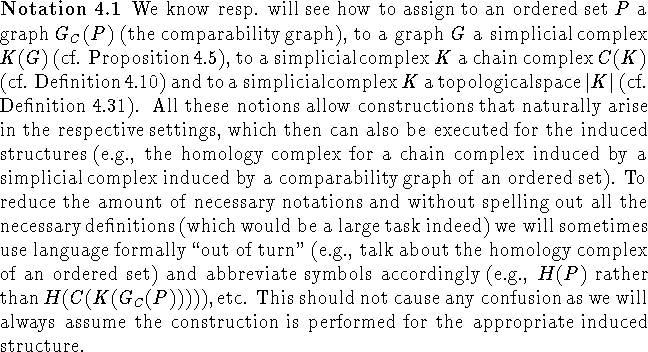Next: Simplicial Complexes Up: Algorithms for the Previous: Isotone Relations

# Order vs. Algebraic Topology

In this section we present the basics of algebraic topology and how they can be used to derive fixed point results for ordered sets. In particular Theorem 4.25 is a homological analogue of Theorem 3.5, leading to a possible similar reduction algorithm as discussed in section 3.2 in order to compute the homology for some ordered sets. This is inspired by the work of Constantin and Fournier (cf. ) discussed in sections 4.5-4.7. We start this section with a review of the basic notions of algebraic topology that are needed here. To keep the necessary new vocabulary limited we will not use the language of category theory here, though those versed in it will easily be able to identify the functors etc. behind the results. Most of the (standard) concepts from algebraic topology have been taken from , chapter 4, sections 1-4. Proofs that were omitted are either short, or a reference to the proof in the literature is given.Bernd.S.W.Schroder